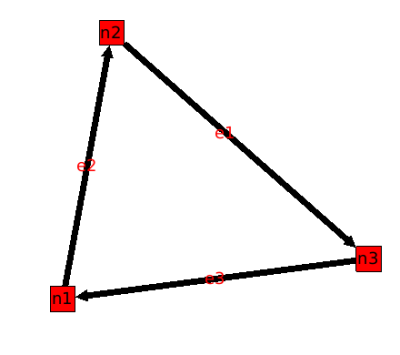## 7. TUTORIAL 001 : Graphs creation, adding and deleting nodes or edges.

In this first tutorial, we will show you how to create a graph, add three nodes and three edges, remove an edge and a node, and finally, print the result on the standard output.```#include <iostream>
#include <tulip/Graph.h>
```

• `iostream :` This "file" contains the C++ standard declarations for in and out streams. We need it in this tutorial to print the final graph on the standard output.

• `tulip/Graph.h :` This file is the core of the tulip graph API. It provides declarations for graphs (edges , nodes) and functions to load one from a file, to save one, and a lot more. You can find a list in the Tulip Graph library documentation.

The namespaces usage declarations, so that we can omit namespace prefix.

```using namespace std;
using namespace tlp;
```

### 2. Creation of a Graph

Create an empty graph with the function ` Graph* tlp::newGraph( )`. This function returns a pointer on a empty Graph.

```int main() {
//create an empty graph
Graph *graph = tlp::newGraph();
```

In the following, we are adding three nodes with the member function ` node Graph::addNode () ` that will, create an instance of a 'node', add it to the graph, and return it.

### Note

Using this function, the node is also added in all the graph ancestors to maintain the sub-graph relation between graphs.

```  //add three nodes
```

Now that nodes are created, we can create the edges. To do so, we can use the function ` edge Graph::addEdge ( const node, const node ) ` that will, add a new edge in the graph and return it.

### Note

The edge is also added in all the super-graph of the graph to maintain the sub-graph relation between graphs.

The first parameter is the "source node", and, of course, the second is the "target node" (in tulip, every edge are oriented but you can choose not to consider the orientation). We will see later (TUTORIAL 005) that the edges enumeration order is the one in which they are added.

```  //add three edges
```

Following is a picture of the graph that we just have created. It is being displayed with tulip.### 5. Delete an edge and a node

The Graph class provides member functions to delete edges and nodes.

• ` void tlp::Graph::delEdge (const edge) ` : delete an edge of the graph. This edge is also removed in all the sub-graphs hierarchy to maintain the sub-graph relation between graphs. The ordering of edges is preserved.

• ` void tlp::Graph::delNode ( const node ) ` : delete a node of the graph. This node is also removed in all the sub-graph of the graph to maintain the sub-graph relation between graphs. When the node is deleted, all its edges are deleted (in and out edges).

### Note

The class Graph implements member functions like `void delAllNode (const node)`, and, `void delAllEdge (const edge)`.

```  //delete an edge
graph->delEdge(e1);

//delete a node
graph->delNode(n2);
```

Following is our graph with node n2 deleted.### 6. Printing the graph

The class graph has a friend function which is an overload of the stream operator <<. This function will print the graph (only nodes and edges) in an output stream (here, the standard output, "cout"), in the tulip format.

```  //print the result on the standard output
cout << graph << flush;
```

### 7. Saving a graph

Instead of having our graph printed on the standard output, we can save it in a .tlp (tulip format) suffixed file that can be read by tulip :

```  //Save  the graph :
tlp::saveGraph(graph,"tuto1.tlp");
```

### 8. Graph deletion

Before exiting the main function, do not forget memory leaks, and delete the graph to free memory usages.

```  //delete the graph
delete graph;
return EXIT_SUCCESS;
}
```

### 9. Compiling and running the program.

Compile this program with this command :

`g++ `tulip-config --libs --cxxflags` tutorial.cpp -o tutorial001 `

Run it to have a look :

` ./tutorial001 `### 10. Source Code

```#include <iostream>
#include <tulip/Graph.h>

/**
*
* Tutorial 001
*
* Create a graph
* add three nodes and three edges
* remove an edge and a node
* print the result on the standard output
*
*/

using namespace std;
using namespace tlp;

int main() {
//create an empty graph
Graph *graph = tlp::newGraph();

//delete an edge
graph->delEdge(e1);

//delete a node
graph->delNode(n2);

//print the result on the standard output
cout << graph << flush ;

tlp::saveGraph(graph,"tuto1.tlp");

//delete the graph
delete graph;
return EXIT_SUCCESS;
}
```